# Contextfree Grammars Section 2 1 more powerful than

• Slides: 11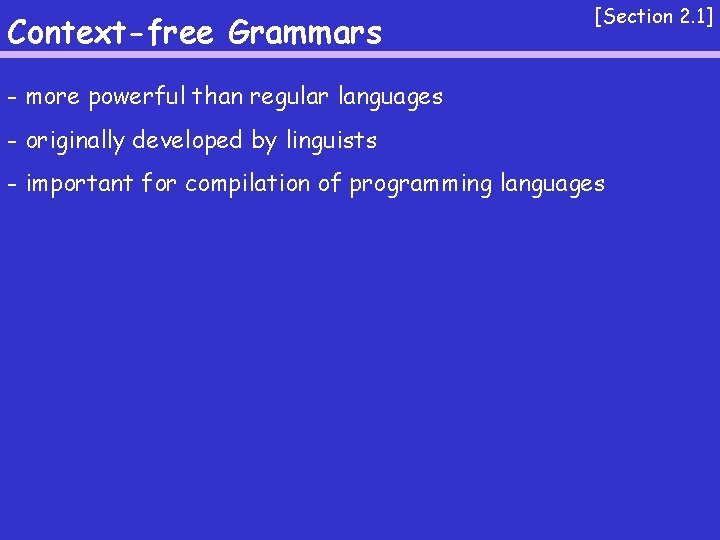Context-free Grammars [Section 2. 1] - more powerful than regular languages - originally developed by linguists - important for compilation of programming languages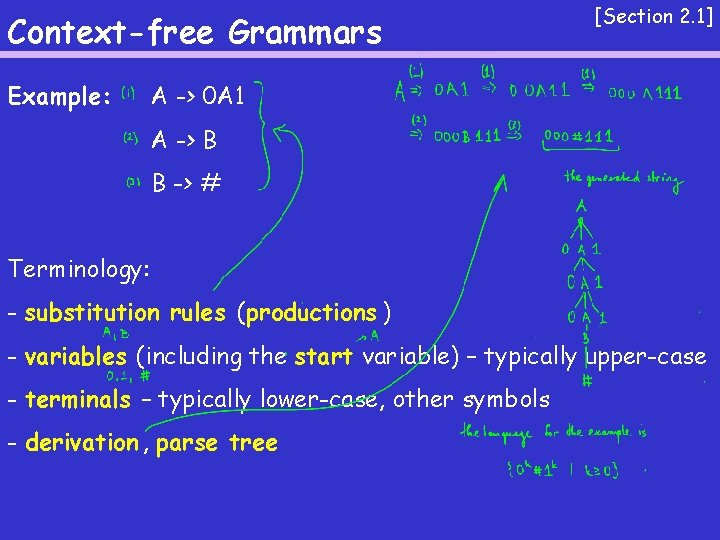Context-free Grammars Example: [Section 2. 1] A -> 0 A 1 A -> B B -> # Terminology: - substitution rules (productions ) - variables (including the start variable) – typically upper-case - terminals – typically lower-case, other symbols - derivation , parse tree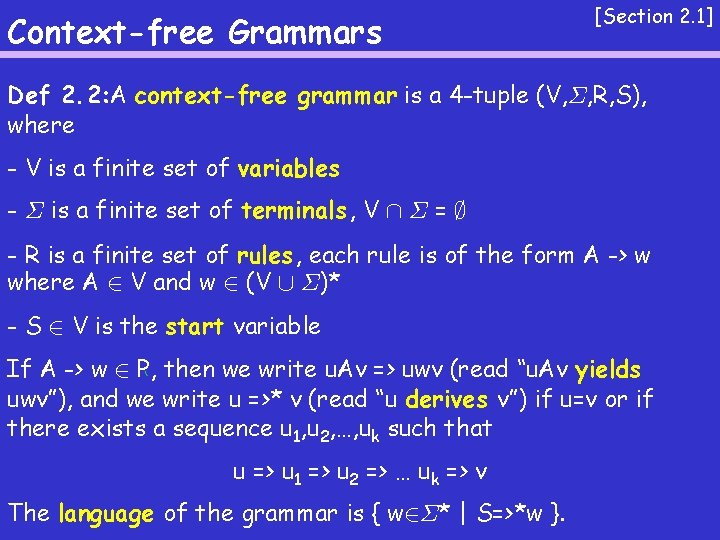Context-free Grammars [Section 2. 1] Def 2. 2: A context-free grammar is a 4 -tuple (V, §, R, S), where - V is a finite set of variables - § is a finite set of terminals , V Å § = ; - R is a finite set of rules, each rule is of the form A -> w where A 2 V and w 2 (V [ §)* - S 2 V is the start variable If A -> w 2 P, then we write u. Av => uwv (read “u. Av yields uwv”), and we write u =>* v (read “u derives v”) if u=v or if there exists a sequence u 1, u 2, …, uk such that u => u 1 => u 2 => … uk => v The language of the grammar is { w 2§* | S=>*w }.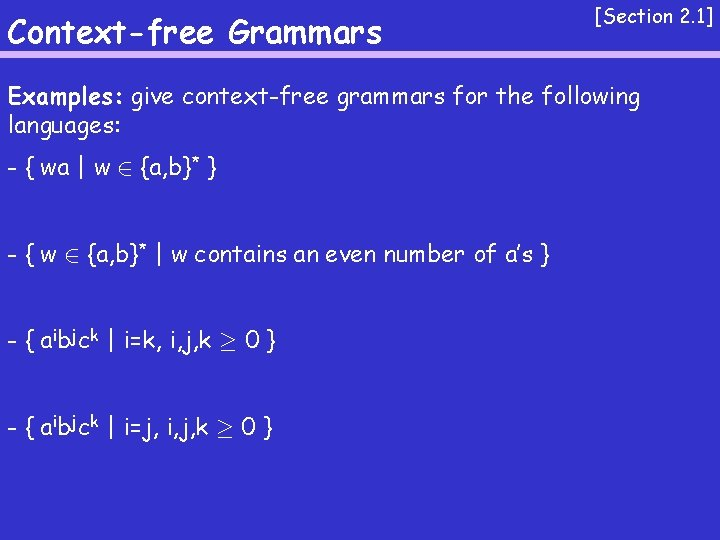Context-free Grammars [Section 2. 1] Examples: give context-free grammars for the following languages: - { wa | w 2 {a, b}* } - { w 2 {a, b}* | w contains an even number of a’s } - { aibjck | i=k, i, j, k ¸ 0 } - { aibjck | i=j, i, j, k ¸ 0 }Context-free Grammars [Section 2. 1] Examples: give context-free grammars for the following languages: - { aibjck | i+j=k, i, j, k ¸ 0 } - strings over { ( , ) } that are well-parenthesized - strings over { 0, 1 } that contain equal number of 0’s and 1’s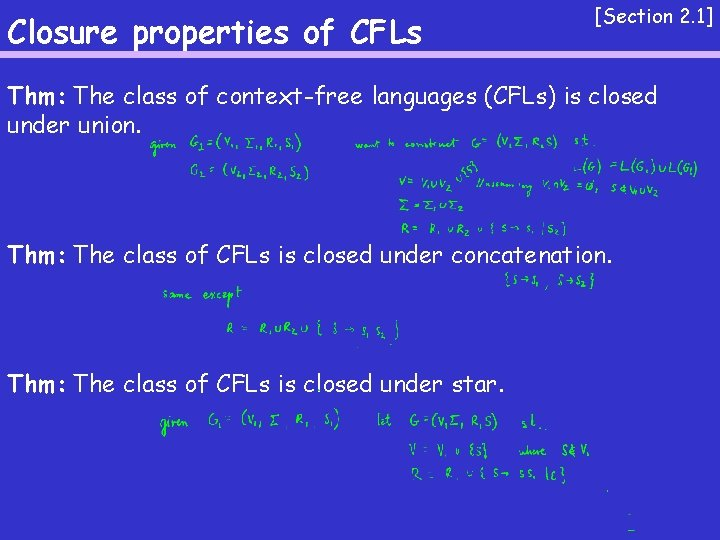Closure properties of CFLs [Section 2. 1] Thm: The class of context-free languages (CFLs) is closed under union. Thm: The class of CFLs is closed under concatenation. Thm: The class of CFLs is closed under star.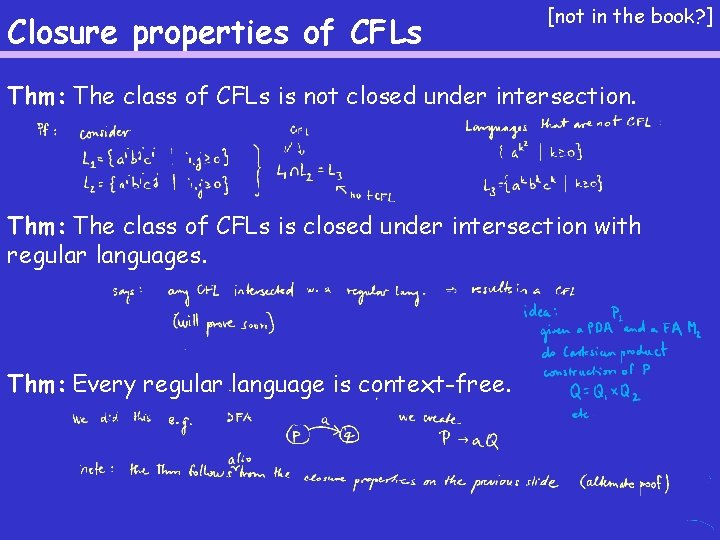Closure properties of CFLs [not in the book? ] Thm: The class of CFLs is not closed under intersection. Thm: The class of CFLs is closed under intersection with regular languages. Thm: Every regular language is context-free.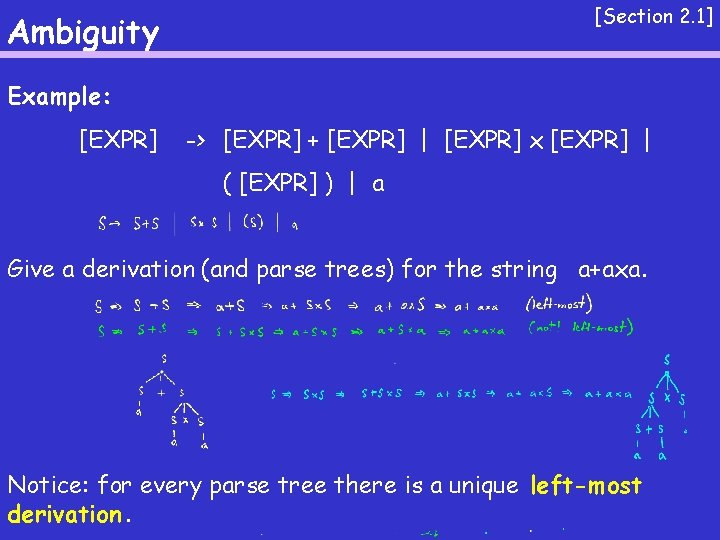[Section 2. 1] Ambiguity Example: [EXPR] -> [EXPR] + [EXPR] | [EXPR] x [EXPR] | ( [EXPR] ) | a Give a derivation (and parse trees) for the string a+axa. Notice: for every parse tree there is a unique left-most derivation.Ambiguity [Section 2. 1] Def 2. 7: A context-free grammar is called ambiguous if there exists a string that can be generated by two different leftmost derivations. Note: Some context-free languages do not have unambigous grammars (e. g { aibjck | i=j or j=k } ). These are called inherently ambiguous. Example: give an unambigous CFG for the language of arithmetic expressions over { +, x, a }Chomsky Normal Form [Section 2. 1] Def 2. 8: A CFG is in Chomsky normal form if every rule is of the form A -> BC or A -> a, where B, C 2 V-{S}, and a 2 §. Thm 2. 9: Any CFL can be generated by a CFG in Chomsky normal form. Note: what about CFL that contains ε ? Why a normal form ?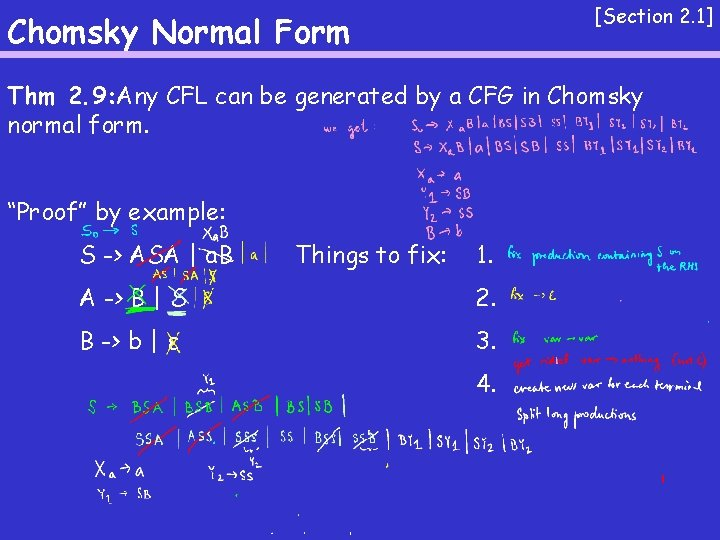[Section 2. 1] Chomsky Normal Form Thm 2. 9: Any CFL can be generated by a CFG in Chomsky normal form. “Proof” by example: S -> ASA | a. B Things to fix: 1. A -> B | S 2. B -> b | ε 3. 4.